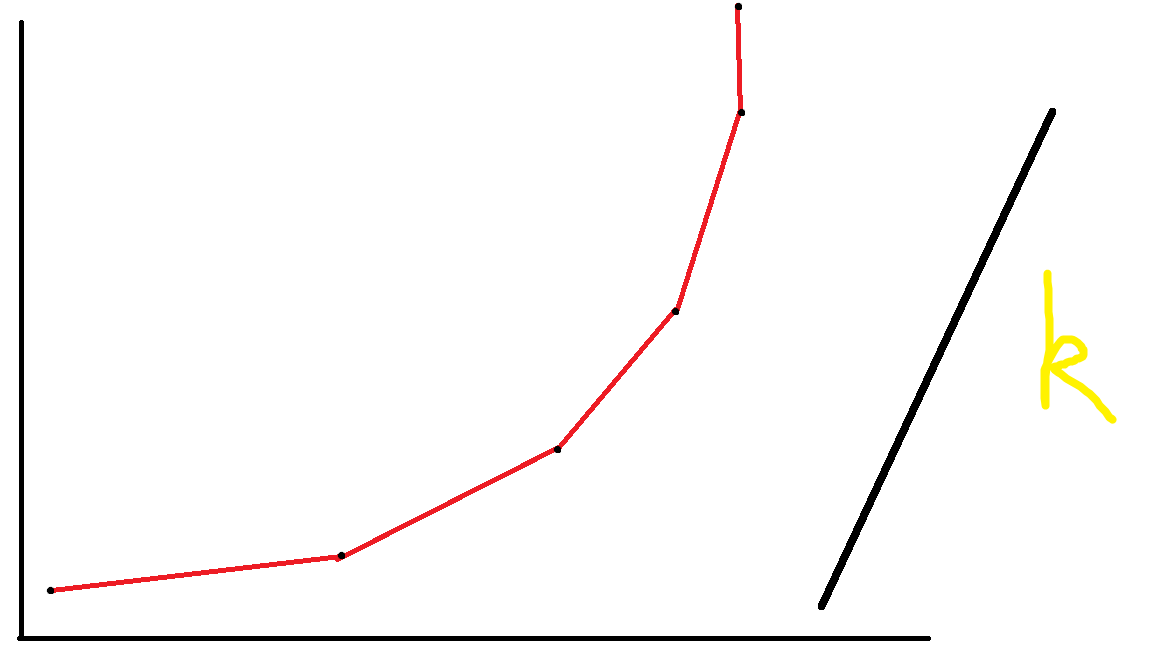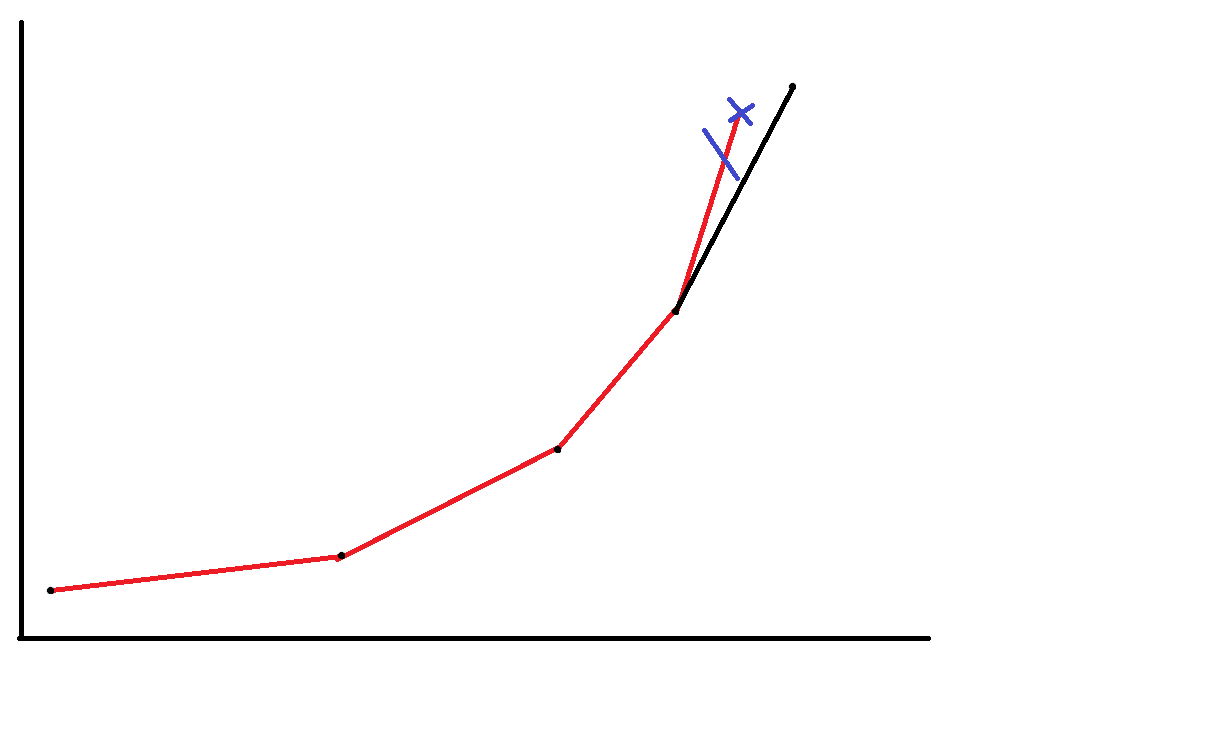# 详解斜率优化

08/15 07:58

dp（废话）

60% n<=1000

100% n<=1000000

额，说了一大坨，大概意思就是说每个点要不花费c[i]直接复制，要不找到后面最近的是直接花费的j，然后花费j-i来复制。

f[i]=a[i]+min{f[j]+(i-j)*(i-j-1)/2}（0<=j<i）

f[i]=a[i]+min{f[j]+(i^2+j^2-2ij-i+j)/2}f[i]=a[i]+min{f[j]+(i-j)*(i-j-1)/2}（0<=j<i）

dp是上面那玩意

f[i]=a[i]+min{f[j]+(i^2+j^2-2ij-i+j)/2}

f[i]-a[i]-(i^2+i)/2=f[j]+(j^2+j-2ij)/2

-f[j]-(j^2-j)/2=-2ij/2

f[j]-(j^2-j)/2=ij

``````#include<cstdio>
#include<cstring>
#include<iostream>
using namespace std;
int n,q;
long long a,f;
double xl(int i,int j){//这个函数是返回斜率滴，返回i与j两个点之间的斜率，由于i与j的横纵坐标都有了，所以就可以求啦。
return ((double)f[i]+(double)i*i/2+(double)i/2-((double)f[j]+(double)j*j/2+(double)j/2))/(i-j);//额这里无脑double了，然后/(i-j)之前一大坨就是纵坐标的差，（i-j）是横坐标的差，相除就是斜率
}
int main(){
scanf("%d",&n);
for(int i=1;i<=n;i++)
scanf("%d",&a[i]);
int h=1,t=1;//头与尾
q=0;//0入队for(int i=1;i<=n;i++){
while(h<t&&xl(q[h],q[h+1])<=i) h++;//当我们还有点可以删的时候，并且此时第一个点与第二个点的斜率<=i，那么就删。　　　　 //首先为什么<=i呢，因为上面我们已经设k=i了啊，换句话说i就是斜率啊。上面的过程就是在原有的点中，找斜率为i的直线的最先遇到的点，而这个点也就是最优决策点。　　　　 //另外再说一下为什么斜率小于（等于时删不删其实无所谓的，因为该删时就一起删，不删时也就选一个）就直接删掉，由于i（也就是斜率）是不断递增的，所以这个点的斜率都小于i了，也必然小于后面的点的斜率。
f[i]=f[q[h]]+a[i]+(i-q[h])*(i-q[h]-1)/2;//这时从队首转移就行啦
while(h<t&&xl(q[t-1],q[t])>=xl(q[t],i)) t--;//这个就是第二个操作了，由于这个时候f求出来了，那么i这个点的横纵坐标就都有了（j，f[j]-(j^2-j)/2），然后做第二个问题就ok啦
q[++t]=i; //i记得加入哦
}
printf("%lld\n", f[n]);
}``````

1.设出动态转移方程

2.通过方程求得坐标以及斜率

3.for循环

4.对于点i，求i的斜率去逼近队列里的点，得到第一个符合条件的

5.此刻通过队首转移f[i]

6.得知f[i]（也许还有其他东西，但是反正我们都知道了），也就是i的坐标得知了，然后把i插入队列里，若不满足凸包（单调性）就删队尾，一直删到满足，把i加入队尾。

1.对于不同的题目，不同的方程，其坐标以及斜率是不一样的（显然），如本题就是i，其他题也许就是2i之类的。

2.对于每道题，我们要考虑其斜率的单调性如何，是单调递增还是单调递减，如本题就是递增。若是递减的话，对于删头尾的操作也有些不同。

1.在方程中，只关于i的或者什么都不关于的我们选择无视，因为这相当于常量，几何意义上属于截距。

2.然后剩下的只有跟j有关和与j和i都有关了。

3.把只与j有关移项

4.这时就发现与j有关的在一边（一坨加减法），与ij有关的（单项式，只有乘）

5.然后只与j有关的是纵坐标，与ij有关的中，i一类为斜率，j一类为横坐标，关于系数尽量归为斜率部分。

关于时间复杂度

1到n遍历O（n），每个节点入队一次出队一次又是O（n），所以总复杂度就是O（n）+O（n）=O（n）（大家都知道啦

0
0 收藏

### 作者的其它热门文章0 评论
0 收藏
0